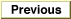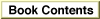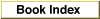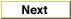# Legacy DocumentImportant: The information in this document is obsolete and should not be used for new development.

Inside Macintosh: QuickDraw GX Environment and Utilities /
Chapter 8 - QuickDraw GX Mathematics / QuickDraw GX Mathematics Reference
Mathematical Functions / Fixed-Point Operations

### FractDivide

You can use the `FractDivide` function to return the quotient of a dividend and divisor.

```fract FractDivide (fract dividend, fract divisor);
```
`dividend`
The number to be divided.
`divisor`
The number by which the dividend is to be divided.
function result
The quotient of two numbers.
##### DESCRIPTION
The `FractDivide` function divides the `dividend` parameter by the `divisor` parameter and returns the quotient. If the `dividend` parameter is a and the `divisor` parameter is b, the quotient a / b is returned.

The format of the number returned depends on the respective number formats of the dividend and divisor. The operation has a bias of 30 bits; in general, the bias of the resulting number is the difference between the biases of the input numbers, shifted left by 30 bits. Thus if the divisor is a `fract`, the result is the same format as the dividend. If the `divisor` and the `dividend` parameters are the same format, the result is in `fract` format, as shown inTable 8-6. The dashed line indicates that the resulting bias is not equivalent to long, fixed, or fract. Use the rules of the operation to determine it
`FractDivide` result bias
DenominatorNumerator
longfixedfract
longfract------
fixed---fract---
fractlongfixedfixed
.

##### SPECIAL CONSIDERATIONS
In the case of division of a large number by a very small number,the `FractDivide` function pins its result to either the `gxPositiveInfinity` or the `gxNegativeInfinity` constant.